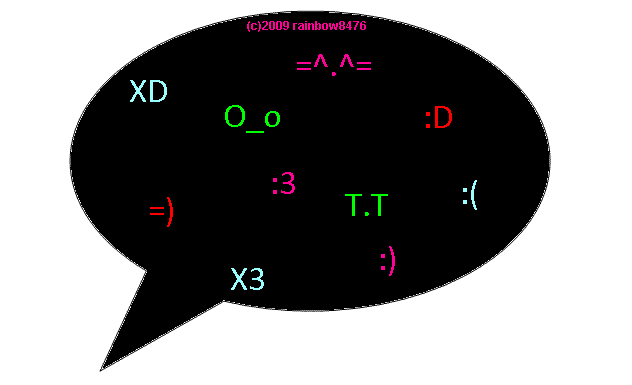Grade 8 Mathematics resources guide will give you a background of Ontario Grade 8 curriculum expectations, resource material, and links for homework help.

## VLC Bookmark

Logins for all VLC databases are found on the back of the VLC Bookmark.## Top picks: Check out these help videos## What do we learn in Number Sense and Numeration?1. Representing and ordering rational numbers;
2. Representing numbers using exponential notation;
3. Solving multi-step problems involving whole numbers and decimals;
4. Multiplying and dividing fractions and integers;
5. Multiplying and dividing decimals by powers of ten;
6. Applying order of operations in expressions with brackets and exponents;
7. Solving problems involving percents to one decimal place and percents greater than 100;
8. Solving problems involving rates and proportions.